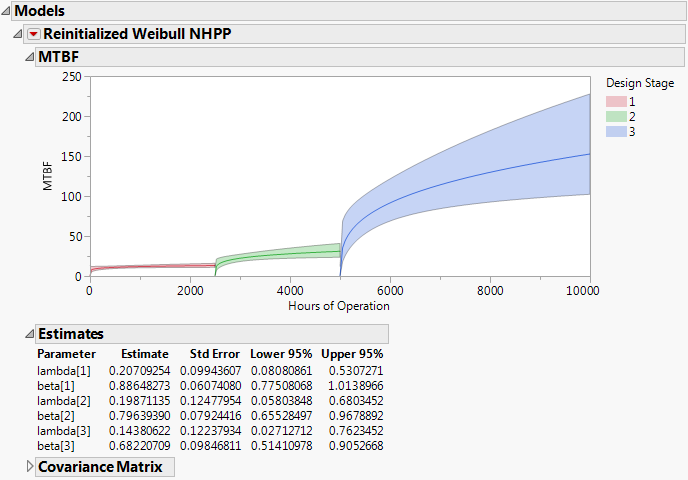Publication date: 05/24/2021

## Reinitialized Weibull NHPP

This option fits an independent growth model to the data from each test phase. Fitting models in this fashion can be useful when the factors influencing the growth rate, either in terms of testing or engineering, have changed substantially between phases. In such a situation, you might want to compare the test phases independently. The Reinitialized Weibull NHPP option is available when a Phase column specifying at least two phases has been entered in the launch window.

For the algorithm to fit this model, each row that contains the first occurrence of a new phase must contain the start date.

Suppose that a single column is entered as Time to Event or Timestamp. Then the start time for a new phase, with a zero Event Count, must appear in the first row for that phase. See the sample data table ProductionEquipment.jmp, in the Reliability subfolder, for an example.

If two columns are entered, then an interval whose left endpoint is that start time must appear in the first row, with the appropriate event count. The sample data table TurbineEngineDesign2.jmp, found in the Reliability subfolder, provides an example. Also, see Piecewise NHPP Weibull Model Fitting with Interval-Censored Data.

Independent Crow-AMSAA models are fit to the data from each of the phases. When the report is run, the Cumulative Events plot updates to show the reinitialized models. Blue vertical dashed lines show the transition points for each of the phases. The Model List also updates.

### Reinitialized Weibull NHPP Report

By default, the Reinitialized Weibull NHPP report shows the estimated MTBF plot. The phases are differentiated with different colors. The Estimates and Covariance Matrix reports are shown below the plot. (See Figure 10.21, which uses the ProductionEquipment.jmp sample data file from the Reliability subfolder.)

Figure 10.21 Reinitialized Weibull NHPP Report#### MTBF Plot

The MTBF plot opens by default when the Reinitialized Weibull NHPP option is chosen. For more information s about the plot, see MTBF Plot.

#### Estimates

The Estimates report gives estimates of λ and β for each of the phases. For a given phase, λ and β are estimated using only the data from that phase. The calculations assume that the phase begins at time 0 and reflect whether the phase is failure or time terminated, as defined by the data table structure. See Test Phases. Also shown are standard errors and 95% confidence limits. These values are computed as described in Parameter Estimates for Crow-AMSAA Models.

#### Covariance Matrix

Estimated covariance matrix for the estimates of the parameters of the fitted model. This report is closed by default.

### Reinitialized Weibull NHPP Options

This section describes the options available in the Reinitialized Weibull NHPP red triangle menu.

#### Show MTBF Plot

This option shows or hides the MTBF plot. See MTBF Plot.

#### Show Intensity Plot

The Intensity plot shows the estimated intensity functions for the phases, along with confidence bands. Because the intensity functions are computed based only on the data within a phase, they are discontinuous at phase transitions. Color coding facilitates differentiation of phases. See Show Intensity Plot.

#### Show Cumulative Events Plot

The Cumulative Events plot for the Reinitialized Weibull NHPP model portrays the estimated cumulative number of events, with confidence bounds, over the design phases in the following way. Let t represent the time since the first phase of testing began. The model for the phase that is in effect at time t is evaluated at time t. In particular, the model for the phase that is in effect is not evaluated at the time since the beginning of the specific phase; rather it is evaluated at the time since the beginning of the first phase of testing.

At phase transitions, the cumulative events functions are discontinuous. The Cumulative Events plot matches the estimated cumulative number of events at the beginning of one phase to the cumulative number at the end of the previous phase. This matching allows the user to compare the observed cumulative events to the estimated cumulative events functions. Color coding facilitates differentiation of phases.

#### Show Profilers

Three profilers are displayed, showing estimated MTBF, Failure Intensity, and Cumulative Events. Note that the Cumulative Events Profiler is constructed as described in the Cumulative Events Plot section. In particular, the cumulative number of events at the beginning of one phase is matched to the number at the end of the previous phase. See Show Profilers.